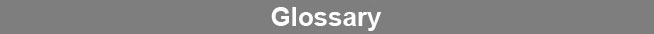Select Page### Variance Equation

A variance equation describes the average variance of adjusted response points as a function of the mean of those responses. The variance equation is computed from a regression of the means and variances of the adjusted responses from the weighting assays. The variance equation is used to weight internal regressions, precision probabilities and residual probabilities.www.brendan.com